/  Technology   /  Bias – Variance Tradeoff in Machine Learning-An Excellent Guide for Beginners!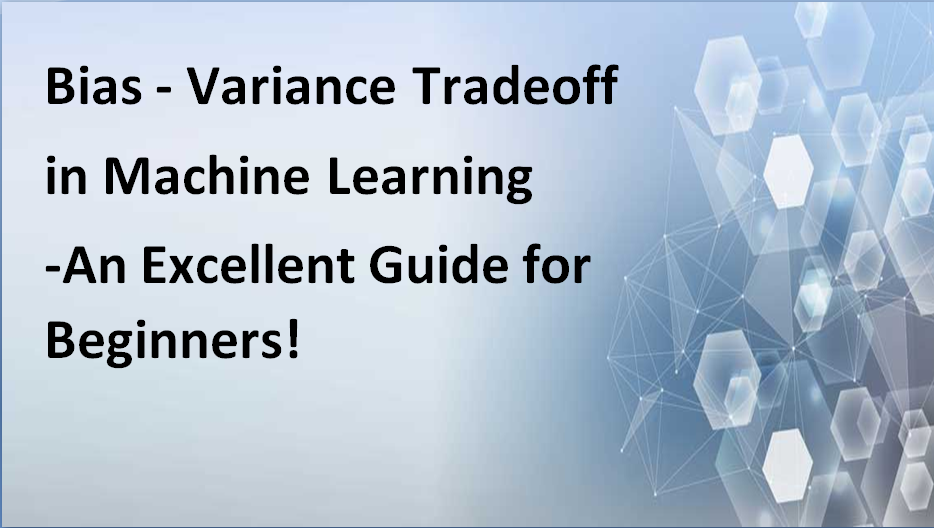## Bias – Variance Tradeoff in Machine Learning-An Excellent Guide for Beginners!

A supervised Machine Learning model aims to train itself on the input variables(X) in such a way that the predicted values(Y) are as near to the actual values as possible. This difference between the actual values and predicted values is the error and it is used to evaluate the model. The error for any supervised Machine Learning algorithm comprises of 3 parts:

1. Bias error
2. Variance error
3. The noise

While the noise is the irreducible error that we cannot eliminate, the other two i.e. Bias and Variance are reducible errors that we can attempt to minimize as much as possible.

There is a tradeoff between a model’s ability to diminish bias and variance. Gaining an appropriate understanding of these errors would help us not only to create accurate models but also to avoid the fault of overfitting and underfitting.

What is Bias?

In the simplest terms, Bias is the difference between the Predicted Value and the Expected Value. Being high in biasing gives a large error in training as well as testing data. Its recommended that an algorithm should be low biased to avoid the problem of underfitting. In supervised learning, underfitting happens when a model unable to capture the underlying pattern of the info. These models usually have high bias.  It happens once we have very less amount of data to create an accurate model or once we try to build a linear model with a nonlinear data. Refer to the graph given below for an example of such a situation.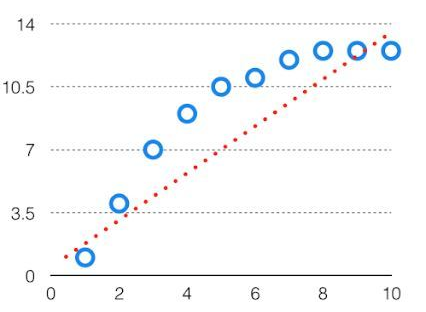In such a problem, a hypothesis looks like follows.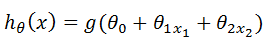What is a Variance?

Variance is that the variability of model prediction for a given data point or a value which tells us spread of our data. Model with high variance has a very complex fit to training data and does not generalize on the data which it hasn’t seen before. As a result, such models do very well on training data but has high error rates on test data. When a model is high on variance, it is then said to as Overfitting of Data. In supervised learning, overfitting happens when our model captures the noise alongside the underlying pattern in data. It happens once we train our model a lot over noisy dataset. These models have high variance. These models are very complex like Decision trees which are susceptible to overfitting.

While training a data model variance should be kept low.

The high variance data looks like follows.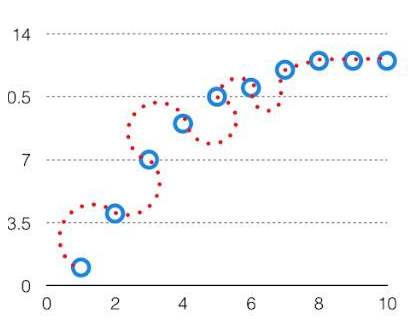In such a problem, a hypothesis looks like follows.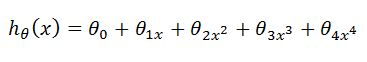If the algorithm is too simple (hypothesis with linear eq.) then it may be on high bias and low variance condition and thus is error-prone. If algorithms fit too complex (hypothesis with high degree eq.) then it may be on high variance and low bias. The balance between the Bias error and the Variance error is the Bias-Variance Tradeoff.

The following bulls-eye diagram explains the tradeoff better: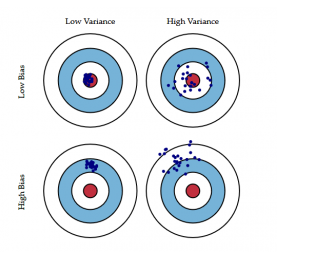The center i.e. the bull’s eye is the model result we want to achieve that perfectly predicts all the values correctly. As we move away from the bull’s eye, our model starts to make more and more wrong predictions.

An algorithm can’t be more complex and fewer complex at the equal time. For the graph, the perfect tradeoff will be like.To build a decent model, we should find out a good balance between bias and variance such that it minimizes the whole error.

Total Error = Bias^2 + Variance + Irreducible Error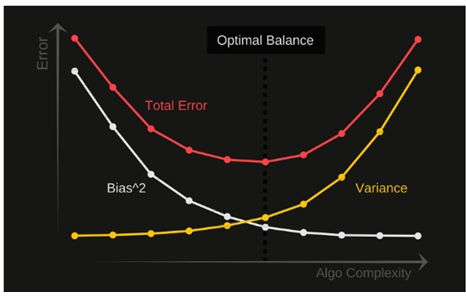An ideal balance of bias and variance would never overfit or underfit the model.

Therefore, understanding bias and variance is critical for understanding the performance of prediction models.